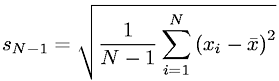Equations > Statistics > Moments > Unbiased Estimator of Populatoin Sample Variance

### Unbiased Estimator of Populatoin Sample VarianceLatex Code:

MathML Code:

 ${s}_{N-1}=\sqrt{\frac1N-1\sum _{i=1}^{N}{\left({x}_{i}-\stackrel{‾}{x}\right)}^{2}}$

MathType 5.0: